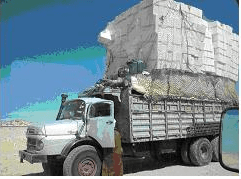# Test: Work And Energy

## 10 Questions MCQ Test Physics For JEE | Test: Work And Energy

Description
This mock test of Test: Work And Energy for JEE helps you for every JEE entrance exam. This contains 10 Multiple Choice Questions for JEE Test: Work And Energy (mcq) to study with solutions a complete question bank. The solved questions answers in this Test: Work And Energy quiz give you a good mix of easy questions and tough questions. JEE students definitely take this Test: Work And Energy exercise for a better result in the exam. You can find other Test: Work And Energy extra questions, long questions & short questions for JEE on EduRev as well by searching above.
QUESTION: 1

### When a body falls freely under gravity, then the work done by the gravity is

Solution:

If a force acting on a body has a component in the direction of displacement, then the work done by the force is positive. Hence when a body falls freely under the influence of gravity the work done by the gravity is positive.

QUESTION: 2

### There are two bodies X and Y with equal kinetic energy but different masses m and 4m respectively. The ratio of their linear momentum is-

Solution:

If X and Y have equal kinetic energy but their masses are m and 4m respectively. Thus we get the ratio of their speed is 2:1. Hence the ratio of their linear momentum is 1:2.

QUESTION: 3

### Ram and sham together can complete a vocation in 8 days. Ram can do likewise work all alone in 12 days. To what extent will sham take to carry out the occupation without anyone else's input?

Solution:

(Ram + sham)'s 1 day work = 1 / 8,
Ram 1 day work = 1 / 12
Sham 1 day work = (1 / 8 - 1 / 12) = 1 / 24
∴ Sham alone can finish the work in 24 days.

QUESTION: 4

A truck moves at a steady speed on a horizontal road. What is the work done by the road on the truck?Solution:

The work is zero because while the truck does have a displacement over time, the force of the road (the normal force) is perpendicular and so the dot product of the two is zero.

QUESTION: 5

Which of the following is not a unit of energy?

Solution:

Energy The joule ( symbol: J) is a derived unit of energy in the International System of Units. It is equal to the energy transferred to (or work done on) an object when a force of one newton acts on that object in the direction of its motion through a distance of one metre (1 newton metreor N⋅m).

QUESTION: 6

Which of the following statements is false:

Solution:

We know that KE = ½ mv2
And as neither the mass nor v2 can be negative.
We get KE cant be negative.

QUESTION: 7

Raindrops fall on the earth with certain kinetic energy on the earth. From where do they get this energy?

Solution:

Every object that tends to fall or does fall on earth's surface does have a gravitational acceleration which is possessed by them due to their gravitational potential energy.

QUESTION: 8

Time rate at which work is done by a force is

Solution:

We know that Work done, W = F.s where F is force and s is displacement due to that force
Thus rate of work done = dW/dt = d(F.s)/dt
So as F is constant we get  dW/dt = F.d(s)/dt
= F.v = P

QUESTION: 9

Output of a truck is 4500 J and its efficiency is 50%, the input energy provided to the truck is

Solution:

Changes(if required):
Solution:
η=50%= 50/100=1/2
So as we know, η=work output/heat input​
1/2=4500/Heat input
Heat input =9000J

QUESTION: 10

When a body slides against a rough horizontal surface, the work done by friction is

Solution:

If a force acting on a body has a component in the opposite direction of displacement, the work done is negative, when a body slides against a rough horizontal surface, its displacement is opposite to that of the force of friction. He work done by the friction is negative.